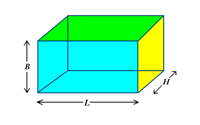# What is volumetric weight?

Volumetric weight is a figure that that takes into account the volume of a package rather than simply the dead weight.
Express international services will travel by air where the volume a package takes up is as important as the weight.
Volumetric weight is necessary since a package with a low weight to volume ratio (for example a small and light item packed in a large box with plenty of space) will cost more to ship than a heavier but denser item that takes less space.

The higher of the dead weight or volumetric weight is used, and the volumetric weight is calculated as below:

Volumetric Weight:
Breadth x Length x Height in centimetres / 5000 = Volumetric Weight in kilograms.So for example if you had a box 50CM by 30CM by 80 CM the volumetric weight would be:

50 X 30 X 80 = 120,000
120,000 / 5000 = 24 KG

So even if in this example the actual weight of the box was 15KG, the chargeable weight would be 24KG.